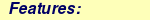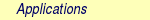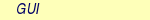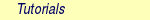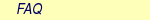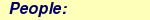MOLCAS manual:Next: 8.42 rpa Up: 8. Programs Previous: 8.40 rasscf

Subsections

# 8.41 rassi

The RASSI (RAS State Interaction) program forms overlaps and other matrix elements of the Hamiltonian and other operators over a wave function basis, which consists of RASSCF wave functions, each with an individual set of orbitals. It is extensively used for computing dipole oscillator strengths, but any one-electron operator, for which the Seward has computed integrals to the ORDINT file, can be used, not just dipole moment components.

Also, it solves the Schrödinger equation projected on the space spanned by these wave functions, i.e., it forms non-interacting linear combinations of the input state functions, and computes matrix elements over the resulting eigenbasis as well.

Finally, using these spin-free eigenstates as a basis, it can compute spin-orbit interaction matrix elements, diagonalize the resulting matrix, and compute various matrix elements over the resulting set of spin-orbit eigenstates.

If only matrix elements of some one-electron operator(s), such as the dipole transition moments, are required, the calculation of Hamiltonian matrix elements and the transformation to the eigenbasis of this matrix can be skipped. However, if any states have the same symmetry and different orbitals, it is desirable to use the transitions strengths as computed between properly non-interacting and orthonormal states. The reason is that the individually optimized RASSCF states are interacting and non-orthogonal, and the main error in the computed transition matrix elements is the difference in electronic dipole moment times the overlap of any two states involved. For excited states, the overlap is often in the order of 10%.

Please note: Due to the increasing number of calculations done with a hundred input states, or more, there has been a demand to change the output. Until MOLCAS6.2, the default assumption has been to print all expectation values and matrix elements that can be computed from the selection of one-electron integrals. From 6.4, this is requested by keywords, see the keyword list below for XVIN, XVES, XVSO, MEIN, MEES, and MESO.

Apart from computing oscillator strengths, overlaps and Hamiltonian matrix elements can be used to compute electron transfer rates, or to form quasi-diabatic states and reexpress matrix elements over a basis of such states.

The CSF space of a RASSCF wave function is closed under deexcitation. For any given pair of RASSCF wave functions, this is used in the way described in reference  to allow the pair of orbital sets to be transformed to a biorthonormal pair, while simultaneously transforming the CI expansion coefficients so that the wave functions remain unchanged. The basic principles are the same as in the earlier program , but is adapted to allow RASSCF as well as CASSCF wave functions. It uses internally a Slater determinant expansion. It can now use spin-dependent operators, including the AMFI spin-orbit operator, and can compute matrix elements over spin-orbit states, i.e. the eigenstates of the sum of the spin-free hamiltonian and the spin-orbit operator.

One use of the RASSI eigenstates is to resolve ambiguities due to the imperfect description of highly excited states. Association between individually optimized states and the exact electronic eigenstates is often not clear, when the calculation involves several or many excited states. The reason is that the different states each use a different set of orbitals. The State Interaction calculation gives an unambiguous set of non-interacting and orthonormal eigenstates to the projected Schrödinger equation, and also the overlaps between the original RASSCF wave functions and the eigenstates. The latter is a very efficient diagnostic, since it describes the RASSCF states in terms of one single wave-function basis set.

To make the last point clear, assume the following situation: We have performed three RASSCF calculations, one where we optimize for the lowest state, one for the first excited state, and one for the 2nd excited state in the same symmetry. The active orbitals are fairly much mixed around, so a simple inspection of the CI coefficient is insufficient for comparing the states. Assume that for each state, we have calculated the three lowest CI roots. It can now happen, that the 2nd root of each calculation is a fair approximation to the exact 2nd eigenstate, and the same with the 3rd, or possibly that the order gets interchanged in one or two of the calculation. In that case, a RASSI calculation with these 9 states will give three improved solutions close to the original ones, and of course 6 more that are considered to be the removed garbage. The overlaps will confirm that each of the input states consists mainly of one particular out of the three lowest eigenstates. This situation is the one we usually assume, if no further information is available.

However, it happens that the active orbitals of the three calculations do not span approximately the same space. The orbital optimization procedure has made a qualitatively different selection of correlating orbitals for the three different calculation. Then the RASSI calculation may well come out with 4 lowest roots that overlap strongly with the original RASSCF states. This may change the assignments and may also give valuable information about the importance of some state. The natural orbitals of the eigenstates will show that the active space used in the RASSCF was in some way inappropriate.

Another bothersome situation is also solved by the RASSI method. The analysis of the original states in terms of RASSI eigenstates may reveal that the three optimized RASSCF states consists mainly of TWO low RASSI eigenstates! This is because the RASSCF optimization equations are non-linear and may sometimes offer spurious extra solutions. Two of the calculations are in this case to be regarded qualitatively, as two different (local) solutions that approximate (imperfectly) the same excited state. Also in this case, the natural orbitals will probably offer a clue to how to get rid of the problem. Extra solutions rarely occur for low states in CASSCF calculations, provided a generous active space can be afforded. Problems occur when the active space is too small, and in particular with general RASSCF calculations.

A further application is the preparation of a suitable orbital basis for a subsequent CI calculation. Note that such an application also allows the use of badly converged RASSCF wave functions, or of RASSCF wave functions containing multiple minima solutions close to a common exact eigenstate. In effect, the RASSI program cleans up the situation by removing the errors due to bad convergence (pushing the errors into a garbage part of the spectrum). This requires that the set of input states (9 in this example) provides flexibility enough to remove at least a major part of the error. As one would expect, this is usually true: The erratic non-convergent, or the too slowly convergent, error mode is to a large extent spanned by the few lowest RASSCF wave functions.

Finally, there are situations where there is no problem to obtain adiabatic RASSCF solutions, but where it is still imperative to use RASSI natural orbitals in a subsequent CI. Consider the case of transition metal chemistry, where there is in general two or more electronic states involved. These states are supposed to interact strongly, at least within some range of interatomic distances. Here, an MCSCF solution, such as RASSCF, will have at least two very different solutions, one associated with each configuration of the transition metal atom. Using one set of orbitals, one electronic state has a reasonably described potential energy curve, while other states get pushed far up in energy. Using another set of orbitals, another state gets correctly described. In no calculation with a single orbital set do we obtain the avoided crossings, where one switches from one diabatic state to another. The only way to accomplish this is via a RASSI calculation. In this case, it is probably necessary also to shift the energies of the RASSCF states to ensure that the crossing occur at the correct places. The shifts can be determined by correcting the atomic spectrum in the separated-atoms limit.

Note, however, that most of the problems described above can be solved by performing state-averaged RASSCF calculations.

## 8.41.1 Dependencies

The RASSI program needs one or more JOBIPH files produced by the RASSCF program. Also, it needs a ONEINT file from SEWARD, with overlap integrals and any one-electron property integrals for the requested matrix elements. If Hamiltonian matrix elements are used, also the ORDINT file is needed.

## 8.41.2 Files

### 8.41.2.1 Input files

 File Contents ORDINT* Ordered two-electron integral file produced by the SEWARD program. In reality, this is up to 10 files in a multi-file system, named ORDINT, ORDINT1,...,ORDINT9. This is necessary on some platforms in order to store large amounts of data. ONEINT The one-electron integral file from SEWARD JOBnnn A number of JOBIPH files from different RASSCF jobs. An older naming convention assumes file names JOB001, JOB002, etc. for these files. They are automatically linked to default files named $Project.JobIph,$Project.JobIph01, $Project.JobIph02, etc. in directory$WorkDir, unless they already exist as files or links before the program starts. You can set up such links yourself, or else you can specify file names to use by the keyword IPHNames. JOBIPHnn A number of JOBIPH files from different RASSCF jobs. The present naming convention assumes file names JOBIPH, JOBIPH01, etc. for such files, when created by subsequent RASSCF runs, unless other names were specified by input. They are automatically linked to default files named $Project.JobIph,$Project.JobIph01, $Project.JobIph02, etc. in directory$WorkDir, unless they already exist as files or links before the program starts. You can set up such links yourself, or else you can specify file names to use by the keyword IPHNames.

### 8.41.2.2 Output files

 File Contents SIORBnn A number of files containing natural orbitals, (numbered sequentially as SIORB01, SIORB02, etc.) If you do not like these names, you must set up links yourselves, except for the first one: SIORB01 is automatically linked to a default file named $Project.SiOrb in directory$WorkDir, if it does not already exists as a file or a link before the program starts. You must set up the other files yourself. TOFILE This output is only created if TOFIle is given in the input. It will contain the transition density matrix computed by Rassi. Currently, this file is only used as input to QmStat. EIGV Like TOFILE this file is only created if TOFIle is given in the input. It contains auxiliary information that is picked up by QmStat.

## 8.41.3 Input

This section describes the input to the RASSI program in the MOLCAS program system, with the program name:

&RASSI

When a keyword is followed by additional mandatory lines of input, this sequence cannot be interrupted by a comment line. The first 4 characters of keywords are decoded. An unidentified keyword makes the program stop.

### 8.41.3.1 Keywords

 Keyword Meaning CHOInput This marks the start of an input section for modifying the default settings of the Cholesky RASSI. Below follows a description of the associated options. The options may be given in any order, and they are all optional except for ENDChoinput which marks the end of the CHOInput section. NoLK Available only within ChoInput. Deactivates the Local Exchange'' (LK) screening algorithm  in computing the Fock matrix. The loss of speed compared to the default algorithm can be substantial, especially for electron-rich systems. Default is to use LK. DMPK Available only within ChoInput. Modifies the thresholds used in the LK screening. The keyword takes as argument a (double precision) floating point (non-negative) number used as correction factor for the LK screening thresholds. The default value is 1.0d-1. A smaller value results in a slower but more accurate calculation. Note: the default choice of the LK screening thresholds is tailored to achieve as much as possible an accuracy of the RASSI energies consistent with the choice of the Cholesky decomposition threshold. NODEcomposition Available only within ChoInput. The inactive Exchange contribution to the Fock matrix is computed using inactive canonical orbitals instead of (localized) Cholesky MOs''. This choice is effective only in combination with the LK screening. Default is to use Cholesky MOs. Note: the Cholesky MOs in RASSI are computed by decomposing the density type supermatrixwhereis the corresponding canonical MOs matrix for the state A and B.When computing the coupling between 2 different states A and B, only for the first state we use pure Cholesky MOs. The invariance of the Fock matrix is then ensured by rotating the orbitals of B according to the orthogonal matrix defined in A through the Cholesky localization. These orbitals used for B are therefore called pseudo Cholesky MOs''. TIME Activates printing of the timings of each task of the Fock matrix build. Default is to not show these timings. MEMFraction Set the fraction of memory to use as global Cholesky vector buffer. Default: for serial runs 0.0d0; for parallel runs 0.3d0. MEIN Demand for printing matrix elements of all selected one-electron properties, over the input RASSCF wave functions. MEES Demand for printing matrix elements of all selected one-electron properties, over the spin-free eigenstates. MESO Demand for printing matrix elements of all selected one-electron properties, over the spin-orbit states. PROPerty Replace the default selection of one-electron operators, for which matrix elements and expectation values are to be calculated, with a user-supplied list of operators. From the lines following the keyword the selection list is read by the following FORTRAN code: READ(*,*) NPROP,(PNAME(I),ICOMP(I),I=1,NPROP) NPROP is the number of selected properties, PNAME(I) is a character string with the label of this operator on SEWARD's one-electron integral file, and ICOMP(I) is the component number. The default selection is to use dipole and/or velocity integrals, if these are available in the ONEINT file. This choice is replaced by the user-specified choice if the PROP keyword is used. Note that the character strings are read using list directed input and thus must be within single quotes, see sample input below. For a listing of presently available operators, their labels, and component conventions, see SEWARD program description. SOCOupling Enter a positive threshold value. Spin-orbit interaction matrix elements over the spin components of the spin-free eigenstates will be printed, unless smaller than this threshold. The value is given in cm-1 units. The keyword is ignored unless an SO hamiltonian is actually computed. SOPROPerty Enter a user-supplied selection of one-electron operators, for which matrix elements and expectation values are to be calculated over the of spin-orbital eigenstates. This keyword has no effect unless the SPIN keyword has been used. Format: see PROP keyword. SPINorbit Spin-orbit interaction matrix elements will be computed. Provided that the ONEL keyword was not used, the resulting Hamiltonian including the spin-orbit coupling, over a basis consisting of all the spin components of wave functions constructed using the spin-free eigenstates, will be diagonalized. NB: For this keyword to have any effect, the SO integrals must have been computed by SEWARD! See AMFI keyword in SEWARD documentation. ONEL The two-electron integral file will not be accessed. No Hamiltonian matrix elements will be calculated, and only matrix elements for the original RASSCF wave functions will be calculated. ONEE is a valid synonym for this keyword. J-VAlue For spin-orbit calculations with single atoms, only: The output lines with energy for each spin-orbit state will be annotated with the approximate J and Omega quantum numbers. OMEGa For spin-orbit calculations with linear molecules, only: The output lines with energy for each spin-orbit state will be annotated with the approximate Omega quantum number. NROF jobiphs Number of JOBIPH files used as input. This keyword should be followed by the number of states to be read from each JOBIPH. Further, one line per JOBIPH is required with a list of the states to be read from the particular file. See sample input below. Alternatively, the first line can contain the number of JOBIPH used as input followed by the word ALL, indicating that all states will be taken from each file. In this case no further lines are required. For JOBIPH file names, see the Files section. Note: If this keyword is missing, then by default all files named 'JOB001', 'JOB002', etc. will be used, and all states found on these files will be used. IPHNames Followed by one entry for each JOBIPH file to be used, with the name of each file. Note: This keyword presumes that the number of JOBIPH files have already been entered using keyword NROF. For default JOBIPH file names, see the Files section. The names will be truncated to 8 characters and converted to uppercase. SHIFt The next entry or entries gives an energy shift for each wave function, to be added to diagonal elements of the Hamiltonian matrix. This may be necessary e.g. to ensure that an energy crossing occurs where it should. NOTE: The number of states must be known (See keyword NROF) before this input is read. In case the states are not orthonormal, the actual quantity added to the Hamiltonian is 0.5D0*(ESHFT(I)+ESHFT(J))*OVLP(I,J). This is necessary to ensure that the shift does not introduce artificial interactions. SHIFT and HDIAG can be used together. HDIAg The next entry or entries gives an energy for each wave function, to replace the diagonal elements of the Hamiltonian matrix. Non-orthogonality is handled similarly as for the SHIFT keyword. SHIFT and HDIAG can be used together. NATOrb The next entry gives the number of eigenstates, for which natural orbitals will be computed. They will be written, formatted, commented, and followed by natural occupancy numbers, on one file each. For file names, see the Files section. The format allows their use as standard orbital input files to other MOLCAS programs. ORBItals Print out the Molecular Orbitals read from each JOBIPH file. OVERlaps Print out the overlap integrals between the various orbital sets. CIPRint Print out the CI coefficients read from JOBIPH. THRS The next line gives the threshold for printing CI coefficients. The default value is 0.05. DIPR The next entry gives the threshold for printing dipole intensities. Default is 1.0D-5. QIPR The next entry gives the threshold for printing quadrupole intensities. Default is 1.0D-5. Will overwrite any value chosen for dipole intesities. QIALL Print all quadrupole intensities. RFPE RASSI will read from RUNOLD (if not present defaults to RUNFILE) a response field contribution and add it to the Fock matrix. HCOM The spin-free Hamiltonian is computed. HEXT It is read from the following few lines, as a triangular matrix: One element of the first row, two from the next, etc, as list-directed input of reals. HEFF A spin-free effective Hamiltonian is read from JOBIPH instead of being computed. It must have been computed by an earlier program. Presently, this is done by a multi-state calculation using CASPT2. In the future, other programs may add dynamic correlation estimates in a similar way. EJOB The spin-free effective Hamiltonian is assumed to be diagonal, with energies being read from a JOBMIX file from a multi-state CASPT2 calculation. In the future, other programs may add dynamic correlation estimates in a similar way. TOFIle Signals that a set of files with data from Rassi should be created. This keyword is necessary if QmStat is to be run afterwards. XVIN Demand for printing expectation values of all selected one-electron properties, for the input RASSCF wave functions. XVES Demand for printing expectation values of all selected one-electron properties, for the spin-free eigenstates. XVSO Demand for printing expectation values of all selected one-electron properties, for the spin-orbit states. EPRG This computes the g matrix and principal g values for the states lying within the energy range supplied on the next line. A value of 0.0D0 or negative will select only the ground state, a value E will select all states within energy E of the ground state. The states should be ordered by increasing energy in the input. The angular momentum and spin-orbit coupling matrix elements need to be available (use keywords SPIN and PROP). For a more detailed description see ref . MAGN This computes the magnetic moment and magnetic susceptibility. On the next two lines you have to provide the magnetic field and temperature data. On the first line put the number of magnetic field steps, the starting field (in Tesla), size of the steps (in Tesla), and an angular resolution for sampling points in case of powder magnetization (for a value of 0.0d0 the powder magnetization is deactivated). The second line reads the number of temperature steps, the starting temperature (K), and the size of the temperature steps (K). The angular momentum and spin-orbit coupling matrix elements need to be available (use keywords SPIN and PROP). For a more detailed description see ref . HOP Enables a trajectory surface hopping (TSH) algorithm which allow non-adiabatic transitions between electronic states during molecular dynamics simulation with DYNAMIX program. The algorithm computes the scalar product of the amplitudes of different states in two consecutive steps. If the scalar product deviates from the given threshold a transition between the states is invoked by changing the root for the gradient computation. The current implementation is working only with SA-CASSCF. STOVerlaps Computes only the overlaps between the input states. TRACk Tries to follow a particular root during an optimization. Needs two JOBIPH files (see NrOfJobIphs) with the same number of roots. The first file corresponds to the current iteration, the second file is the one from the previous iteration (taken as a reference). With this keyword RASSI selects the root from the first JOBIPH with highest overlap with the root that was selected in the previous iteration. It also needs MDRlxRoot, rather than RlxRoot, to be specified in RASSCF. No other calculations are done by RASSI when Track is specified. DQVD Perfoms DQdiabatization by using properties that are computed with RASSI. One must compute 7 properties with RASSI in order for this keyword to work (x,y,z,xx,yy,zz,1/r), and they must be specified in that order. At present, this keyword also requires ALPHa and BETA, where ALPHa is the parameter in front of rr and BETA is the parameter in front of 1/r. When ALPHa and BETA are equal to zero, this method reduces to Boys localized diabatization.At present, this method only works for one choice of origin for each quantity. ALPHa ALPHa is the prefactor for the quadrupole term in DQdiabatization. This keyword must be used in conjunction with DQVD and BETA. You must specify a real number (e.g.not). BETA BETA is the prefactor for the electrostatic potential term in DQdiabatization. This keyword must be used in conjunction with DQVD and ALPHa. You must specify a real number (e.g.not). TRDI Prints out the components and the module of the transition dipole vector. Only vectors with sizes large than 1.0D-4 a.u. are printed. See also the TDMN keyword. TDMN Prints out the components and the module of the transition dipole vector. On the next line, the minimum size, in a.u., for the dipole vector to be printed must be given.

### 8.41.3.2 Input example

»COPY "Jobiph file 1" JOB001 »COPY "Jobiph file 2" JOB002 »COPY "Jobiph file 3" JOB003

&RASSI
NR  OF  JOBIPHS=  3  4  2  2  --  3  JOBIPHs.  Nr  of  states  from  each.
1  2  3  4;  3  4;  3  4  --  Which  roots  from  each  JOBIPH.
CIPR;  THRS=  0.02
Properties=  4;  'MltPl  1'  1  'MltPl  1'  3  'Velocity'  1  'Velocity'  3
*  This  input  will  compute  eigenstates  in  the  space
*  spanned  by  the  8  input  functions.  Assume  only  the  first
*  4  are  of  interest,  and  we  want  natural  orbitals  out
NATO=  4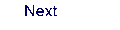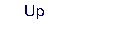Next: 8.42 rpa Up: 8. Programs Previous: 8.40 rasscf